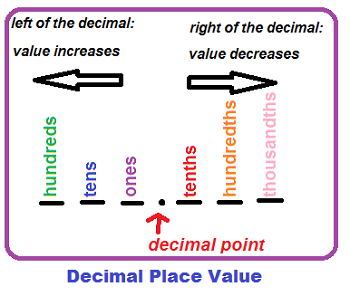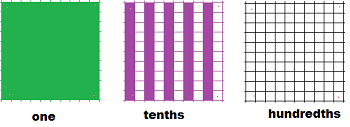# Decimals: Lesson for Kids

Instructor: Bethany Calderwood

Bethany has taught special education in grades PK-5 and has a master's degree in special education.

You've probably used whole numbers in math, but can you write a number that is smaller than one? In this lesson, you will learn how decimals show numbers smaller than one, and you'll practice reading and writing them.

## Numbers That Are Less Than One

When you are working with money, measurement, or statistics, you often have numbers that are smaller than one. How do you write them?

Sometimes, it makes sense to write the number as a fraction. An example of this would be if you cut your pizza into four slices and ate one slice. You ate one out of four pieces of pizza--that's ¼ of the pizza.

In many contexts, fractions are difficult to work with. In these cases, you can use another mathematical tool: the decimal, which represents a part of a whole just like a fraction does. It contains a decimal point, which looks like a period (' . ') and is used in a number to separate the whole number from the part of the number that is less than one.

## Place Value

To fully understand decimals, we must first review the concept of place value. In a multi-digit number, place value tells us how much each digit is worth.When you use a decimal point, the ones, tens, and hundreds places all fall to the left of the decimal point. They all represent whole numbers. As you start at the decimal point and go left, the values of the places grow larger--ones, then tens, then hundreds, then thousands, etc.

Numbers that fall to the right of a decimal point, on the other hand, are all valued at less than one. As you start at the decimal and go right, the values of the places grow smaller. Look at the following decimal numbers:

• In 0.1, the 1 is in the tenths place. It has a value of one-tenth.
• In 0.01, the 1 is in the hundredths place. It has a value of one-hundredth.

When you read a number with a decimal, the decimal point stands for ''and.'' The numbers after the decimal points are read using the place value label of the digit furthest to the right. So, the number 1.5 is said 'one and five-tenths.' The number 2.43 is said 'two and forty-three hundredths.'

## Tenths and Hundredths

You can use blocks to better understand place value. Look at the picture of these three blocks:The green block represents one whole. The number in the ones place represents how many green blocks you have, and in this case that number would be 1.

To unlock this lesson you must be a Study.com Member.

### Register to view this lesson

Are you a student or a teacher?

#### See for yourself why 30 million people use Study.com

##### Become a Study.com member and start learning now.
Back
What teachers are saying about Study.com

### Earning College Credit

Did you know… We have over 200 college courses that prepare you to earn credit by exam that is accepted by over 1,500 colleges and universities. You can test out of the first two years of college and save thousands off your degree. Anyone can earn credit-by-exam regardless of age or education level.